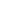#Watt's Law ELE-1011 is a Course

## Watt's Law ELE-1011

Self-paced

### Full course description

Course Description

Watt’s law is the mathematical representation of the relationship between power, current, and voltage in an electrical circuit. Understanding how to apply Watt’s law is important for anyone working with electrical circuits.

By the end of this course, you will be able to

• Define Watt’s law

• Apply Watt’s law to calculate unknown electrical values

• Combine Watt’s law and Ohm’s law to calculate unknown electrical values

Estimated completion time (hours): 0.8